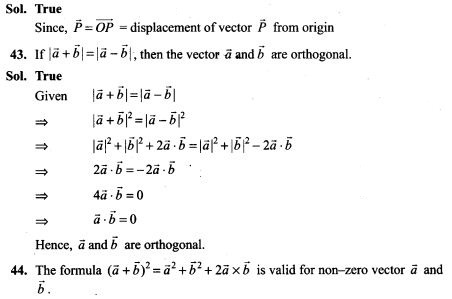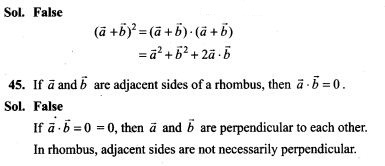# Vector Algebra NCERT Exemplar Problems Solutions Class 12th

0
2043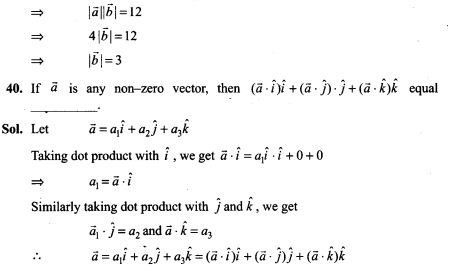## NCERT Exemplar Problems Class 12 Mathematics Chapter 10 Vector Algebra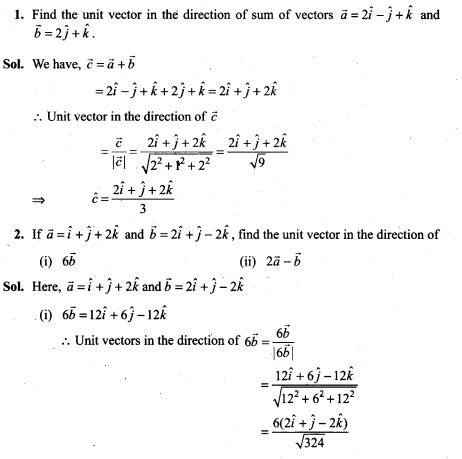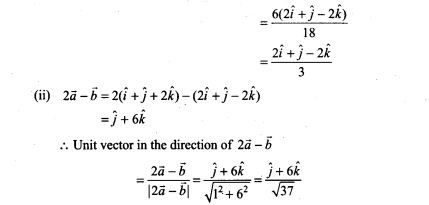3. Find a unit vector in the direction of PQ, where P and Q have co-ordinates (5,0, 8) and (3, 3,2), respectively.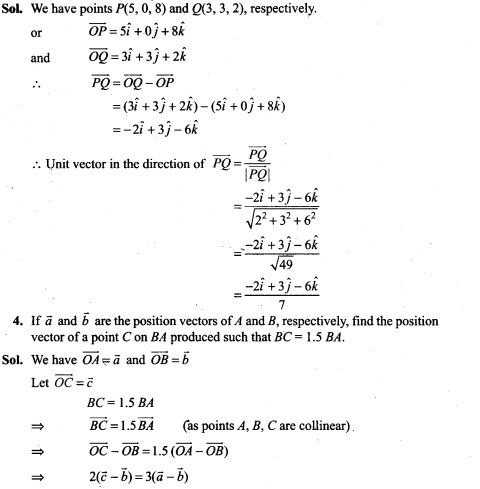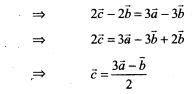5. Using vectors, find the value of k such that the points (k, -10, 3), (1, -1, 3) and (3, 5, 3) are collinear.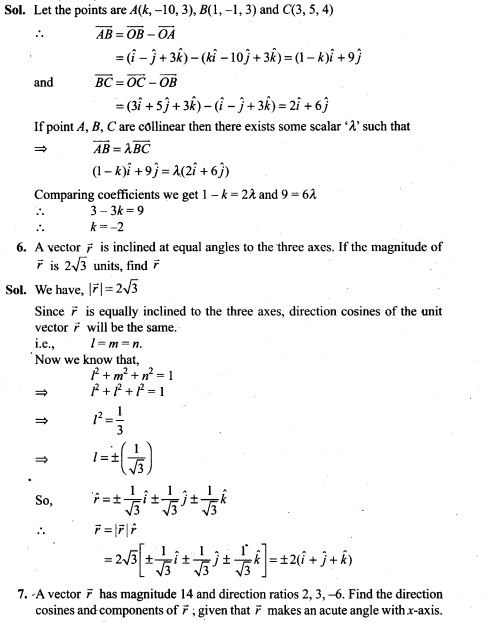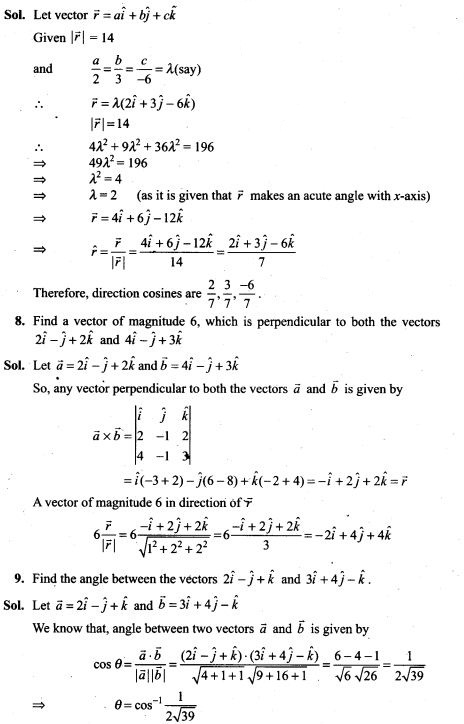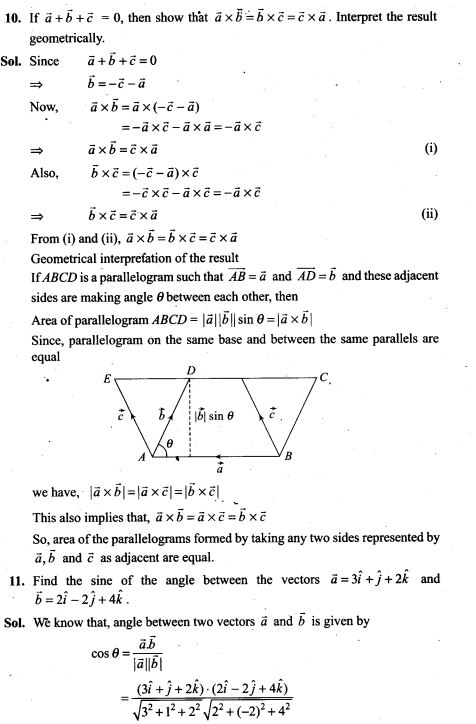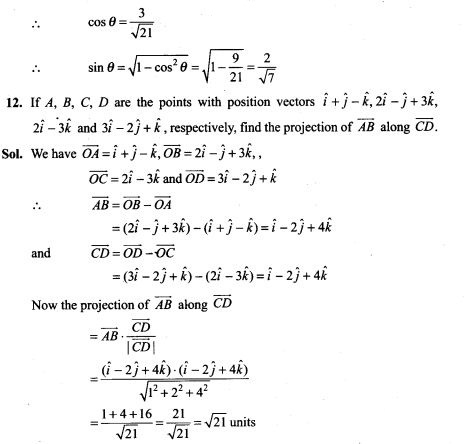13. Using vectors, find the area of the triangle ABC with vertices A(l, 2, 3), 5(2, -1, 4) and C(4,5,-l).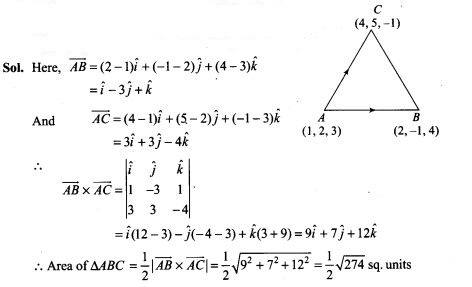14. Using vectors, prove that the parallelogram on the same base and between the same parallels are equal in area.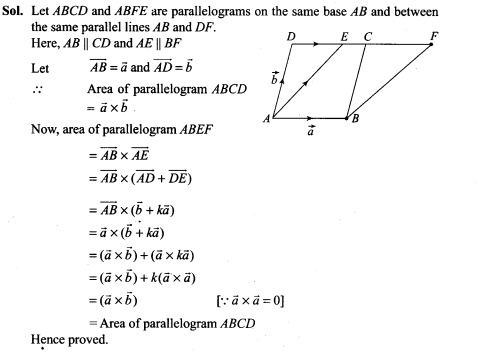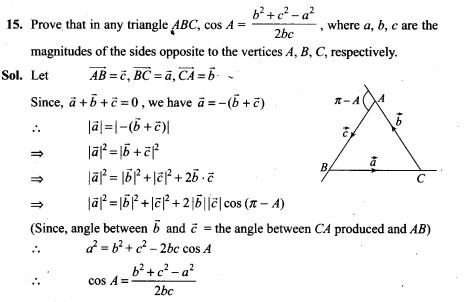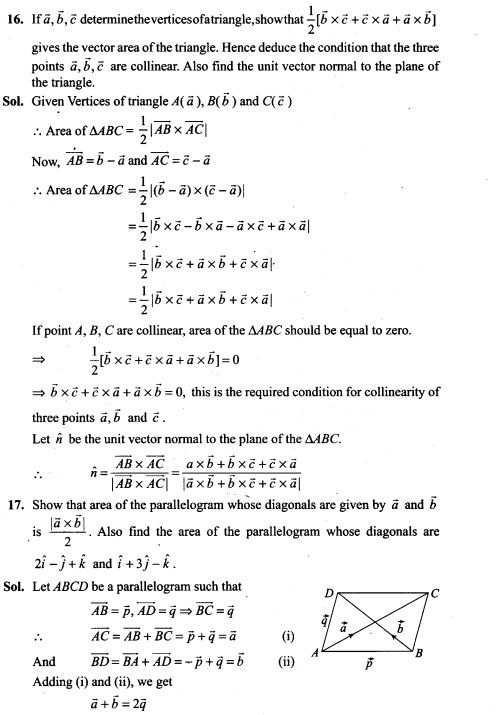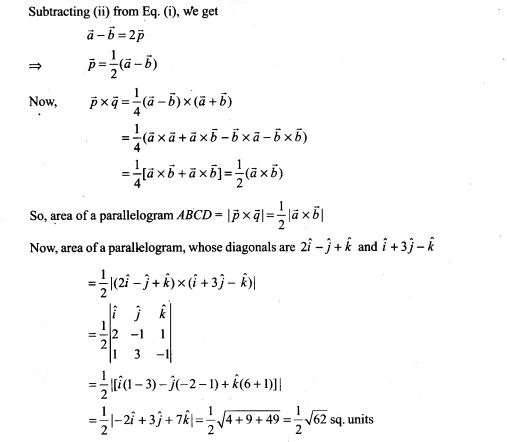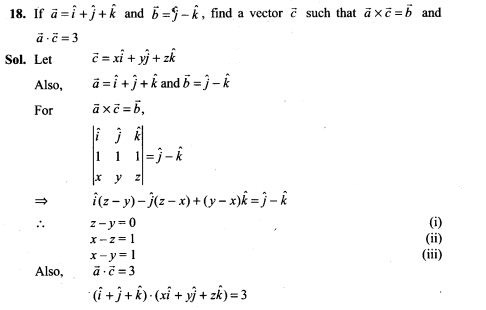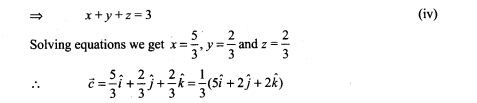Objective Type Questions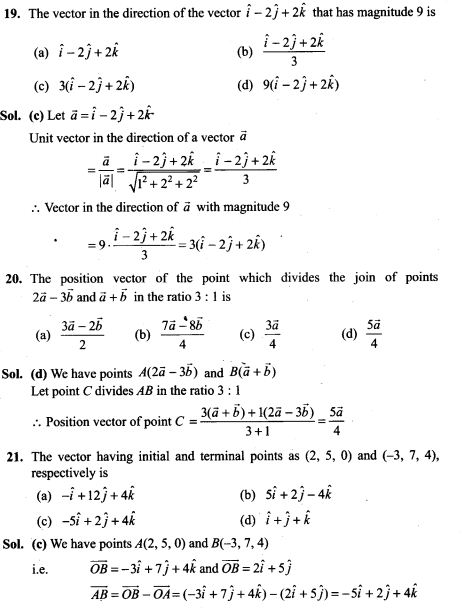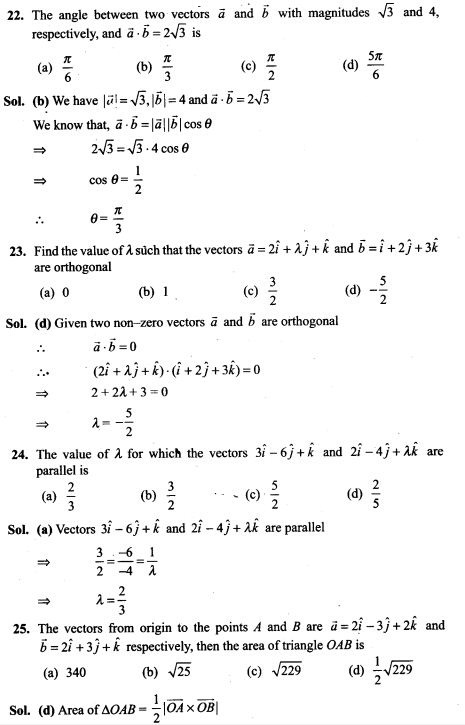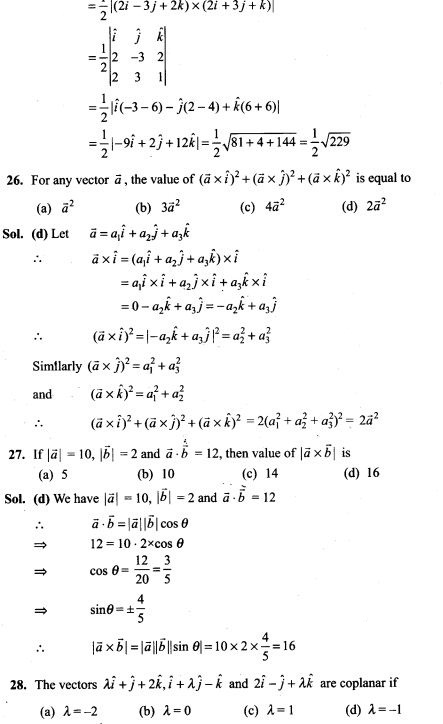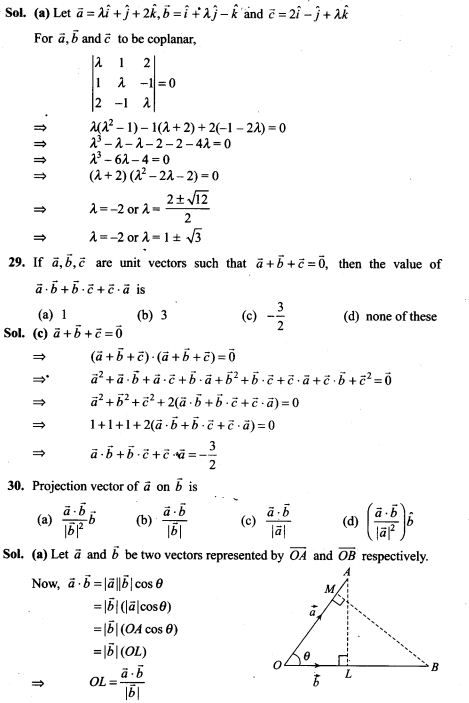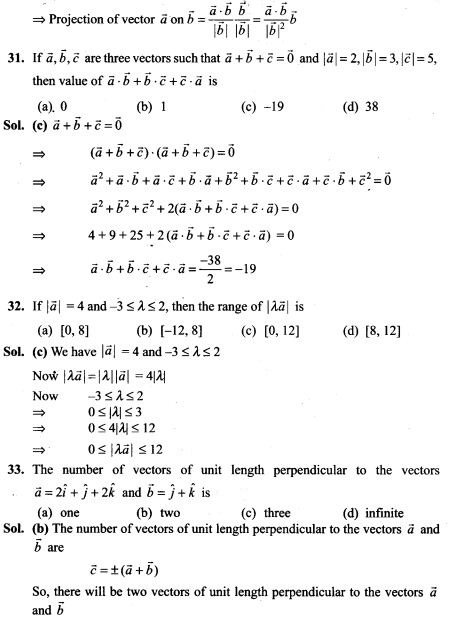Fill in the Blanks Type Questions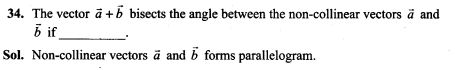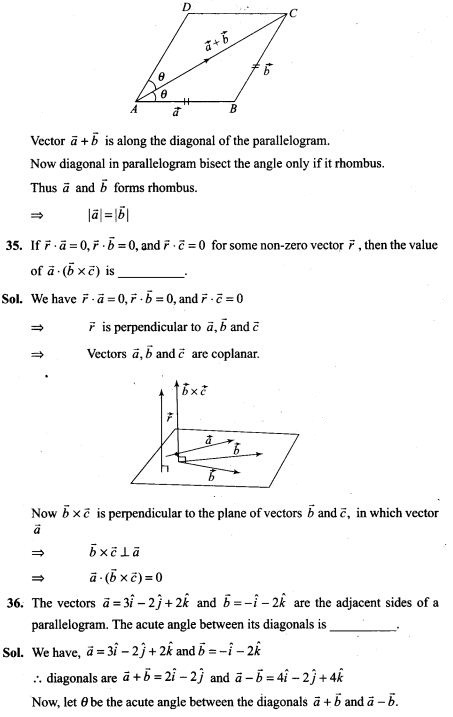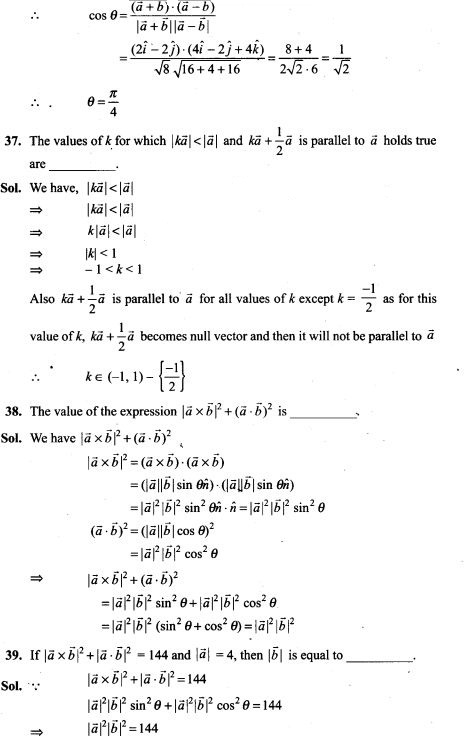True/False Type Questions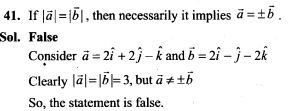42. Position vector of a point P is a vector whose initial point is origin.